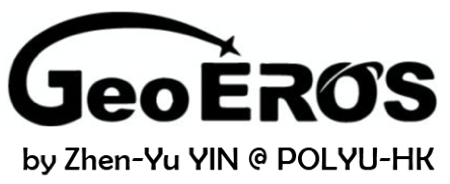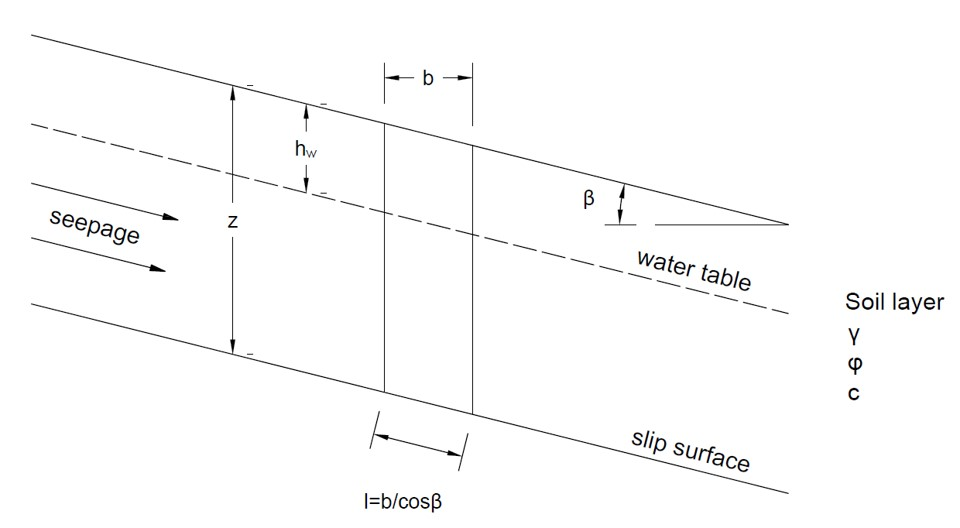Slope Tool (translational sliding)Geometry of Slope Stability (translational sliding)Tips

• This tool is for slope staility analysis where the translational sliding surface is parallel to the slope surface, as shown in the figure above.
• The slip surface and water table are assumed as parallel to the slope surface.
• If water is not considered, hw = z. If soil layer is saturated, hw = 0.
• For cohesionless slope, the cohesive strength c should be 0.
• The minimum safety factor for slope is obtained in the calculation.
• All the input parameters should be positive.
• The unit weight of water is 9.8 kN/m3.
• For "nan", "0" or "inf" displayed in Results, please check your input parameters.

z (m)
hw (m)
β (°)
γ (kN/m3)
c (kN/m2)
φ (°)